• 敏感度分析的基础概念文本主要参考了维基百科（对其中的关键部分进行了摘选了翻译）：https://en.wikipedia.org/wiki/Sensitivity_analysis​en.wikipedia.org敏感度分析是指对一个模型输出中的不确定性进行研究,并...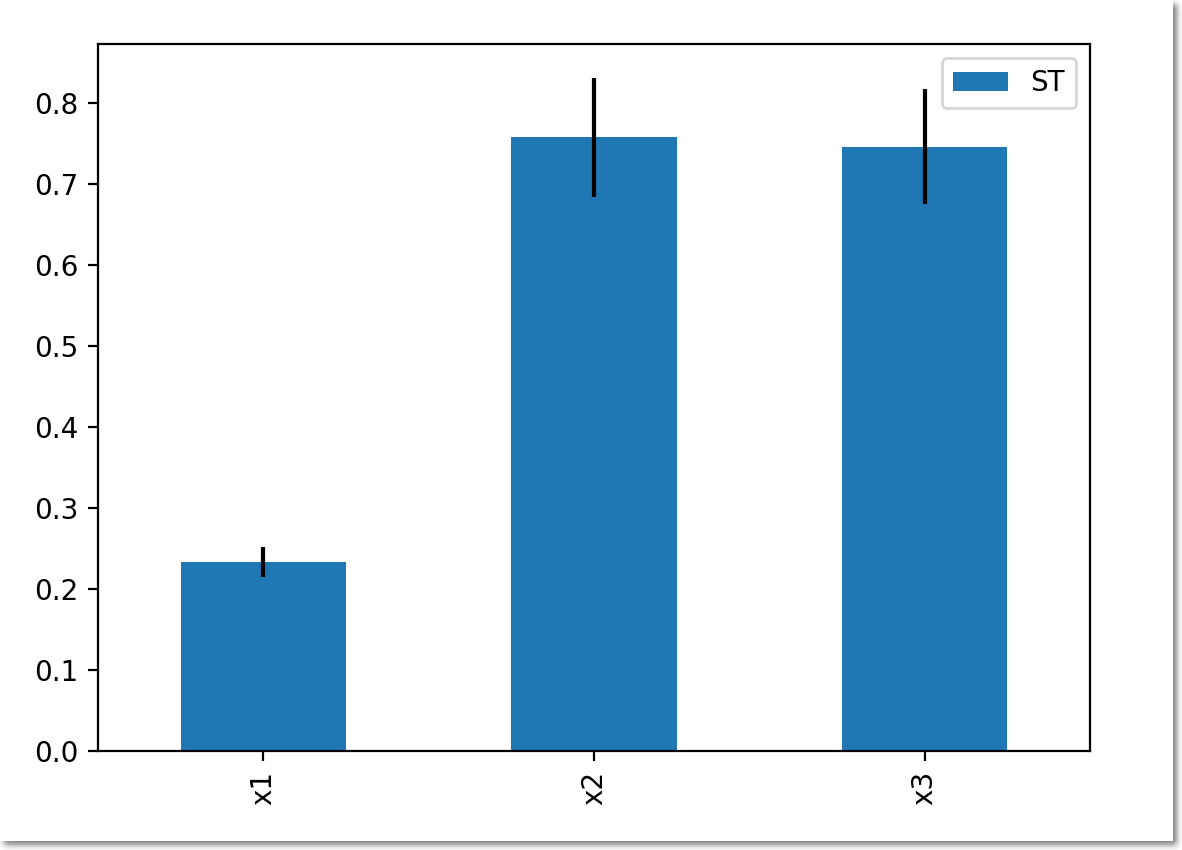敏感度分析的基础概念文本主要参考了维基百科（对其中的关键部分进行了摘选了翻译）：https://en.wikipedia.org/wiki/Sensitivity_analysis​en.wikipedia.org敏感度分析是指对一个模型输出中的不确定性进行研究,并进一步判断不确定性的来源,也就是研究哪个输入参数的改变造成的输出变化的程度大小. 所以灵敏度分析是进行数学建模过程中一个必不可少的常规步骤.选择敏感度分析方法的时候需要考虑的要素：每运行一次模型的计算代价输入参数之间的相关性模型的响应是否非线性输入因素之间的相互作用已有数据的输入范围 常见的敏感度分析方法（跳过了傅里叶分析相关的方法，只有最后两个方法是全局分析）：One at a time(OAT) 方法每次变动一个输入并检查对于输出的影响。这种方法很简单，但由于它没有考虑输入变量的同时变化，因此并未充分探索输入空间。也无法检测输入变量之间是否存在交互。Screening方法窗口法是一种基于采样的方法。目的是要确定哪些输入变量对高维模型中的输出不确定性有重大影响，而不是准确地量化灵敏度。它具有相对较低的计算成本，并且可以在对其余集合应用更具信息性的分析之前，用于初步分析中以清除无影响的变量。最常用的筛选方法之一是基本效应方法（moris方法）散点图法基于偏导数的局部分析法检查输出对于各个输入的偏导数也无法充分探索输入空间Adjoint modelling and Automated Differentiation 都属于这类方法回归分析在敏感性分析的背景下，回归分析包括将线性回归拟合到模型响应，并使用标准化回归系数作为敏感性的直接度量。因此，当模型响应实际上是线性时，此方法最合适。例如，如果确定系数大，则可以确认回归模型有效。回归分析的优点是简单且计算成本低。基于方差的方法（sabol方法）是全局方法。可以处理非线性响应，并且可以度量非加性系统中相互作用的影响。属于概率论方法。可将输入和输出不确定性量化为概率分布，并将输出方差分解为可归因于输入变量和变量组合的部分。因此，输出对输入变量的敏感度通过该输入引起的输出变化量来度量。常常会涉及蒙特卡洛方法 基于响应面的方差分析（VARS）这种通过一系列多个变量、确定性的“试验”，来模拟真实极限状态曲面的方法称为响应面法。  相关软件分析工具的外部链接SimLab，联合研究中心用于全球敏感性分析的免费软件灵敏度分析Excel加载项是一个免费的（供私人和商业使用）Excel加载项，它允许基于样本的简单灵敏度分析运行MUCM项目 –用于计算需求模型的不确定性和敏感性分析的大量资源。GEM-SA –使用高斯过程执行灵敏度分析的程序。Python中的SALib灵敏度分析库（Numpy）。包含Sobol，Morris，分数阶乘和FAST方法。(另外在matlab 和 sas， spss 等商业软件中也都有敏感度分析工具)Python中的敏感度分析python中的敏感度分析工具是salib,可以直接通过pip安装,  而且支持的敏感度分析方法能够满足很多需求. 下面这个例子是我完善了补充了该库官方的入门示例后的小成果, 更加通俗易懂(使用sobol方法分析了模型 y = x+ x* cos(2* x )    的灵敏度):从整体步骤上说, salib先提供采样输入, 拿到采样输入后我们讲其送入我们想要分析的自定义的模型(任何python实现的模型都可以), 然后再将模型的对应输出送回 salib就可以完成分析了.from SALib.sample import saltelli
from SALib.analyze import sobol
import numpy as np
import math

# Define the model inputs
problem = {
'num_vars': 3,
'names': ['x1', 'x2', 'x3'],
'bounds': [[-3.14159265359, 3.14159265359],
[-3.14159265359, 3.14159265359],
[-3.14159265359, 3.14159265359]]
}

def evaluate(X):  # 这里是我们要进行灵敏度分析的模型,接受一个数组,每个数组元素作为模型的一个输入,模型的输出是一个float,干函数返回的时候再讲所有输出并起来
return np.array([math.sin(x) + x * math.cos(2 * x) for x in X])

# Generate samples
param_values = saltelli.sample(problem, 1000)

# Run model (example)
Y = evaluate(param_values)
print(param_values.shape, Y.shape)
# Perform analysis (这里运行完成后会自动对结果进行展示)
Si = sobol.analyze(problem, Y, print_to_console=True)
print()

# Print the first-order sensitivity indices  一阶灵敏度
print('S1:', Si['S1'])

# Print the second-order sensitivity indices   二阶灵敏度
print("x1-x2:", Si['S2'][0, 1])
print("x1-x3:", Si['S2'][0, 2])
print("x2-x3:", Si['S2'][1, 2])该程序的输出如下:(8000, 3) (8000,)

Parameter S1 S1_conf ST ST_conf
x1 0.241379 0.040549 0.233006 0.020597
x2 -0.001635 0.075150 0.757444 0.083538
x3 -0.019499 0.082548 0.746122 0.064596

Parameter_1 Parameter_2 S2 S2_conf
x1 x2 -0.002905 0.057218
x1 x3 -0.007212 0.051962
x2 x3 0.768228 0.138674

S1: [ 0.24137922 -0.00163541 -0.01949871]
x1-x2: -0.0029050009015768627
x1-x3: -0.007212159874777576
x2-x3: 0.7682277244642012输出结果的一般形式：Si是一个字典，含有"S1"， "S2"，"ST"，"S1_conf"，"S2_conf"，和 "ST_conf"项目。其中"S1"， "S2"，"ST" 分别表示一阶灵敏度，二阶灵敏度和总灵敏度。（一个二阶灵敏度会涉及两个变量）, 而对应他们名字加上_conf 则表示对应的置信度（95%）。灵敏度分析结果出现负数，说明灵敏度很小，并且因为样本太小所以出现了一些统计误差。然后我们还能使用salib自带的灵敏度结果可视化功能:from SALib.plotting.bar import plot as barplot
import matplotlib.pyplot as plot

Si_df = Si.to_df()
barplot(Si_df)
plot.show()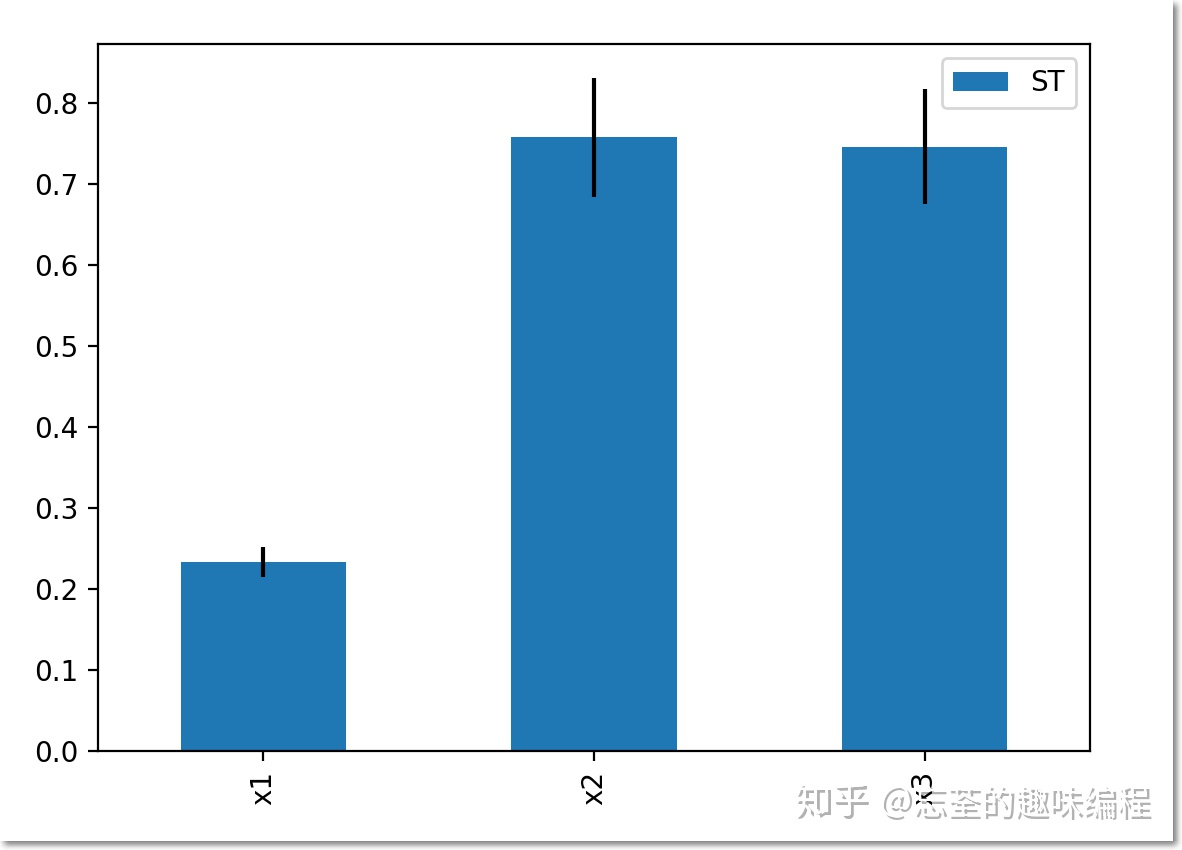模型参数总灵敏度可视化 需要注意的是,原salib文档中的画图说明中有错误, 需要在 Si_df 后面加入一个索引再送入画图函数,原说明文档中缺少了一个索引,所以会出错.
展开全文• 通过计算各个影响因素对输出结果的影响进行灵敏度分析。 假设模型为，为模型中影响因素的个数，每个影响因素的变动范围为其拟合值的5%-20%。假设将其每个影响因素的变化范围映射到区间[0,1]，然后分成N个均匀间隔，...
本文采用Morris方法对参数的全局灵敏度进行评价。通过计算各个影响因素对输出结果的影响进行灵敏度分析。

假设模型为，为模型中影响因素的个数，每个影响因素的变动范围为其拟合值的5%-20%。假设将其每个影响因素的变化范围映射到区间[0,1]，然后分成N个均匀间隔，成为 {0,1/（N-1），...，1}。

为了计算个参数的基本效应，需要（+1）模拟值，其方法与对每个参数扰动的局部灵敏度方法相同，称为一个“路径”。通过随机生成参数集的多个路径，我们得到了每个参数的基本效果的集合。计算总数为，其中r为路径数。

一次路径的步骤如下：

（1）构建×m（m=+1）的矩阵B：

一开始每个参数必须随机从{0，1/（N-1），...，1}中取值作为样本。每个轨迹采样都有一个随机生成的不同起点。在矩阵B中，第1列表示每个参数的样本，相邻两列只有一个参数的取值不同。1表示变化的因素，0表示不变的因素。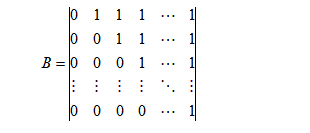（2）一次依次改变一个参数，把矩阵B中相邻两列作为模型的输出参数，即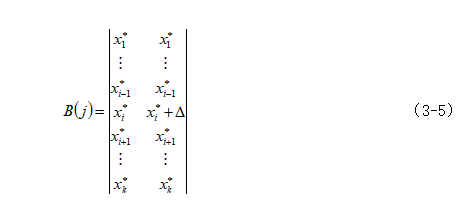式中，这里∆为预先设定的变化量，。由基本效应（EE）来评估，其定义为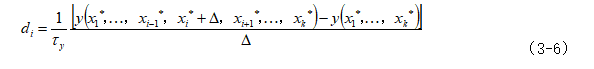其中是输出比例因子，参数集可以通过蒙特卡罗随机采样随机选择。

一次路径结束，重复r次。

本实验的目的是估计初等效应分布的平均值和均方差，这些初等效应分布是用Morris方法的全局灵敏度分析中的r次独立随机路径估计的，平均值和均方差称为第参数的灵敏度因子，可对该参数的灵敏度进行判断：当平均值很小时，则说明该参数对模型的影响很小，该参数不重要；当平均值较大，则说明该参数与模型输出有线性关系；均方差比较小时，则说明该参数与其他参数的相关性弱；但当平均值和均方差都比较大时，则说明该参数与模型输出有非线性关系，并且该参数与其他参数的相关性、关联性强。

代码获取

展开全文python 机器学习 算法 java 人工智能
• 掌握使用matlab、Lingo、Excel的规划求解功能求解，并利用“敏感性报告”进行分析。(二)实验内容课本例1解的灵敏度分析(1)：调用单纯形程序：function[x,z,flg,sgma]=simplexfun(A,A1,b,c,m,n,n1,cb,xx)%A,...
实验二、线性规划的灵敏度分析(一)实验目的1.线性规划求解的单纯形法的灵敏度分析的编程实现2．掌握使用matlab、Lingo、Excel的规划求解功能求解，并利用“敏感性报告”进行分析。(二)实验内容课本例1解的灵敏度分析(1)：调用单纯形程序：function [x,z,flg,sgma]=simplexfun(A,A1,b,c,m,n,n1,cb,xx)% A,b are the matric in A*x=b% c is the matrix in max z=c*x% A1 is the matric in simplex table% m is the numbers of row in A and n is the con number in A%n1isthenubersofartificialvariables,andartificialvariablesaredefaultasthelast%n1variables in x.% cb is the worth coefficient matrix for basic variables% xx is the index matrix for basic variables% B1 is the invers matrix for the basic matrix in simplex table.The initial% matrix is default as the last m con in the matrix A.x=zeros(n,1);z=0;B1=A1(:,n-m+1:n);sgma1=c-(cb*B1)*A;[masg,kk]=max(sgma1);k=kk(1);flg=0;ll=0;while (masg>0)&&(ll<20)ll=ll+1;thita=1000+zeros(m,1);for i=1:mif A1(i,k)>0thita(i)=A1(i,k)\b(i);endend[r8,c8]=find(thita>999);if sum(c8)[mith,rr]=min(thita);r=rr(1);aa=A1(r,k);for i=1:mif i==r
展开全文• 问题是，我想知道对于此数据和模型，参数a,b,c的敏感性，也就是y的改变量与a、b、c的改变量的比值关系。首先用1stopt分析：1 NewCodeBlock"SA";2 Parameter a=[1,3],b=[2,4],c=[3,5];//要优化的参数及其范围3 ...
首先看抛物线函数：现在我取a=2,b=3,c=4 ,得到如下函数：x或t都是指自变量，就不改了，一个意思。问题是，我想知道对于此数据和模型，参数a,b,c的敏感性，也就是y的改变量与a、b、c的改变量的比值关系。首先用1stopt分析：1 NewCodeBlock"SA";2 Parameter a=[1,3],b=[2,4],c=[3,5];//要优化的参数及其范围3 Variable t,y;//变量4 Function y=a*t^2+b*t+c;5 Data;6 //t    y 变量顺序要和Variable后变量对应7 -5    398 -4    249 -3    1310 -2    611 -1    312 0    413 1    914 2    1815 3    3116 4    4817 5    69以下分别为各种参数敏感性方法(包括morris局部和全局，偏微分方法局部和全局)：以上就是4中方法的结果。用的目标函数是SSR,点的范围我用的是上下浮动50%，正好布满整个给定空间：可以看morris局部敏感度分析具体数据：a：b：c：然后将灵敏度指数平均得到morris局部方法(本质是OAT方法，一次改变一个)分析结果：全局方法(本质是通过拉丁抽样实现同时考虑多个参数的影响)就不是这个思路了，看一下超拉丁抽样：总结一下1stopt中4种方法参数灵敏度结果：morris方法局部：名称 灵敏度指数 灵敏度指数(%)a3.916E1889.1113892365457b4.125E179.38673341677096c6.6E161.50187734668335morris方法全局：名称 灵敏度指数 灵敏度指数(%)a2.00290323137447E1881.4890482414753b2.10289523274038E178.55572692595605c2.44687506069835E179.95522483256862偏微分方法局部：名称 灵敏度指数 灵敏度指数(%)a3.99432000000567E1889.1113892365577b4.20750000000213E179.38673341676365c6.73199999998753E161.50187734667864偏微分方法全局：名称 灵敏度指数 灵敏度指数(%)a3.98876054886661E1882.084202339751b4.20542269921853E178.65428655186941c4.50049450186404E179.26151110837963下面用matlab写sobol方法进行分析：1 %sobol 参数敏感性分析2 %算法参考：3 % csdn : https://blog.csdn.net/xiaosebi1111/article/details/465174094 % wiki： https://5 %运行环境 matlab2016b6 %作者 blzhu@buaa.edu.cn 2020年6月7日7 %%初始化8 clc;9 clear all;10 close all;11 %%设定：给定参数个数和各个参数的范围1213 % % 1-自定义子函数114 % D=3;%维度3,几个参数15 % nPop=4:500:5000;%采样点个数,也就是参数水平数 ,取大了好，比如4000，但慢16 % VarMin=[-pi -pi -pi ];%各个参数下限  SALib ：S1: [ 0.30797549  0.44776661 -0.00425452] ；ST: [0.56013728 0.4387225  0.24284474]17 % VarMax=[pi pi pi];%各个参数上限18 % myFunction=@(x) Ishigami(x);%目标函数，也可以是个黑盒子1920 % % 1-自定义子函数221 D=3;%维度3,几个参数22 nPop=4:50:1000;%采样点个数,也就是参数水平数 ,取大了好，比如4000，但慢23 VarMin=[1 2 3 ];%各个参数下限24 VarMax=[3 4 5];%各个参数上限25 myFunction=@(x) myx2(x);2627 % % 1-自定义子函数328 % D=4;%维度3,几个参数29 % nPop=4:50:1000;%采样点个数,也就是参数水平数 ,取大了好，比如4000，但慢30 % VarMin=[1 2 3 4];%各个参数下限31 % VarMax=[3 4 5 5];%各个参数上限32 % myFunction=@(x) myx3(x);333435 %%开始计算36 numnPop=numel(nPop);37 SAll=zeros(numnPop,D+1);%分别是各参数的敏感度，最后一列是各参数敏感度之和38 STAll=zeros(numnPop,D+1);39 for i=1:numnPop40     [S,ST]=sobol(D,nPop(i),VarMin,VarMax,myFunction);41     SAll(i,1:D)=S';42     SAll(i,D+1)=sum(SAll(i,1:D));43     STAll(i,1:D)=ST';44     STAll(i,D+1)=sum(STAll(i,1:D));45 end46 %%绘图47 color=[1 0 0;0 1 0;0 0 1;0.5 0.1 0;0 0.3 0.4;0.6 0.7 0.2;0.5 0.8 0.9;0 0.2 0.1;0.1 0.5 0;0.1 0 0.5;0.5 0 0.1];%12种颜色 一般颜色不一样48 marker=['o','+','*','.','x','s','d','^','v','>','52 figure(1)53 for i=1:D+154 plot(nPop,SAll(:,i),'Marker',marker(i),'LineStyle',char(linestyle(useL)),'Color',color(i,:),'LineWidth',1);55 hold on56 end57 title('Sobol-S');58 whichPara=sprintfc('%g',repmat(1:D+1,1,2));%把数字数组转化成字符串数组59 legend(whichPara,'Location','bestoutside');%加图例606162 figure(2)63 for i=1:D+164 plot(nPop,STAll(:,i),'Marker',marker(i),'LineStyle',char(linestyle(useL)),'Color',color(i,:),'LineWidth',1);65 hold on66 end67 title('Sobol-ST');68 whichPara=sprintfc('%g',repmat(1:D+1,1,2));%把数字数组转化成字符串数组69 legend(whichPara,'Location','bestoutside');%加图例7071 disp('一阶影响指数(左方向收敛于1)Sobol-S：');72 disp(S);73 disp('总效应指数(大于等于1，且仅当myfun是纯相加时取等号)Sobol-ST：');74 disp(ST);75 disp(datetime);76 disp('parameter sensitive analyse success use sobol method!');77 %%火车声音提示已经算完了78 load train79 sound(y,Fs)80818283 %% -------------------------子函数 matlab2016之前不支持子函数写在同一个m文档中----------------------------84 % 1-自定义子函数1(3个参数)Ishigami  https://www.sfu.ca/~ssurjano/ishigami.html85 function y=Ishigami(x)86 y=sin(x(1))+7*(sin(x(2)))^2+0.1*x(3)^4*sin(x(1));%SALib用的这个87 % y=sin(x(1))+7*(sin(x(2)))^2+0.05*x(3)^4*sin(x(1));88 end8990 % 1-自定义子函数2 (3个参数)91 function y=myx2(x)92 t=-5:1:5;%与此处有t范围和步距有关系93 % t=-5:0.1:5;%与此处有t范围和步距有关系94 ylab=2*t.^2+3*t+4;95 ytheory=x(1)*t.^2+x(2)*t+x(3);96 y=sum((ylab-ytheory).^2);%残差平方和SSR作为目标函数97 % y=sum((ylab-ytheory).^2)/numel(t);%各参数灵敏度与上式相同98 end99100101 % 1-自定义子函数3(4个参数)102 function y=myx3(x)103 t=-5:1:5;104 ylab=2*t.^3+3*t.^2+4*t+5;105 ytheory=x(1)*t.^3+x(2)*t.^2+x(3)*t+x(4);106 y=sum((ylab-ytheory).^2);107 end108109110111 %% 2-求sobol敏感度112 function [S,ST]=sobol(D,nPop,VarMin,VarMax,myFunction)113 M=D*2;%114 %%产生所需的各水平参数115 VarMin=[VarMin,VarMin];116 VarMax=[VarMax,VarMax];117 p= sobolset(M);% https://www.cnblogs.com/zhubinglong/p/12260292.html118 % R=p(1:nPop,:);%我只用前nPop个119 R=[];120 for i=1:nPop121     r=p(i,:);122     r=VarMin+r.*(VarMax-VarMin);123     R=[R; r];124 end125 % plot(R(:,1),'b*')126 %拆分为A B127 A=R(:,1:D);%每行代表一组参数，其中每列代表每组参数的一个参数；行数就代表共有几组参数128 B=R(:,D+1:end);129 %根据A B 产生矩阵AB130 AB=zeros(nPop,D,D);131 for i=1:D132     tempA=A;133     tempA(:,i)=B(:,i);134     AB(1:nPop,1:D,i)=tempA;135 end136 %%求各参数解137 YA=zeros(nPop,1);%解138 YB=zeros(nPop,1);139 YAB=zeros(nPop,D);%分别代表YAB1,YAB2,YAB3，YAB(:,D)就代表YABD140 for i=1:nPop141     YA(i)=myFunction(A(i,:));142     YB(i)=myFunction(B(i,:));143     for j=1:D144         YAB(i,j)=myFunction(AB(i,:,j));145 end146 end147 %%根据一阶影响指数公式：148 VarX=zeros(D,1);%S的分子149 S=zeros(D,1);150151 % 0: 估算基于给定样本的方差(EXCEL var.p) ;   1:计算基于给定的样本总体的方差(EXCEL var.p())152 % var([2.091363878    1.110366059    3.507651769    1.310950363    2.091363878    3.507651769    1.110366059    1.7066512],1);153 VarY=var([YA;YB],1,'omitnan');% S的分母。 计算基于给定的样本总体的方差(EXCEL var.p())154 for i=1:D155     for j=1:nPop156          VarX(i)=VarX(i)+YB(j)*(YAB(j,i)-YA(j));157 end158      VarX(i)=1/nPop*VarX(i);%蒙特卡罗估计量159      S(i)=VarX(i)/VarY;160 end161162 %%总效应指数163 EX=zeros(D,1);164 ST=zeros(D,1);165 for i=1:D166     for j=1:nPop167          EX(i)=EX(i)+(YA(j)-YAB(j,i))^2;168 end169       EX(i)=1/(2*nPop)* EX(i);%蒙特卡罗估计量170      ST(i)=EX(i)/VarY;171 end172 end我分别取了不同个数的样点 4:50:1000 ，结果如下，可见1000个样点基本稳定了。各参数的灵敏度：一阶影响指数(左方向收敛于1)Sobol-S：0.97280.00300.0001总效应指数(大于等于1，且仅当myfun是纯相加时取等号)Sobol-ST：0.98600.00310.0155当然，也可以在matlab的fileexchange上下载各种工具箱，但这个根据csdn和wiki上写的算法相对简单些，便于魔改。用python的SALib包分析Method of Morris, including groups and optimal trajectories ([Morris 1991], [Campolongo et al. 2007])Fourier Amplitude Sensitivity Test (FAST) ([Cukier et al. 1973], [Saltelli et al. 1999])Delta Moment-Independent Measure ([Borgonovo 2007], [Plischke et al. 2013])Derivative-based Global Sensitivity Measure (DGSM) ([Sobol and Kucherenko 2009])Fractional Factorial Sensitivity Analysis ([Saltelli et al. 2008])下面以sobol方法举例：1 #https://salib.readthedocs.io/en/latest/basics.html#run-model2 #-*- coding: utf-8 -*-3 from SALib.sample importsaltelli4 from SALib.analyze importsobol5 from SALib.test_functions importIshigami6 importnumpy as np7 importmath8 from SALib.plotting.bar importplot as barplot9 importmatplotlib.pyplot as plot1011 #problem = {12 #'num_vars': 3,13 #'names': ['x1', 'x2', 'x3'],14 #'bounds': [[-3.14159265359, 3.14159265359],15 #[-3.14159265359, 3.14159265359],16 #[-3.14159265359, 3.14159265359]]17 #}1819 problem ={20     'num_vars': 3,21     'names': ['x1', 'x2', 'x3'],22     'bounds': [[1, 3],23                [2, 4],24                [3, 5]]25 }262728 param_values = saltelli.sample(problem, 1000)#不管用哪个方法计算y,这个要有29 np.savetxt("param_values.txt", param_values)#将参数变化保存，其实是各参数范围内的sobol抽样3031 ##  计算Y32 ##1-自定义-133 #Y = np.zeros([param_values.shape])34 #A = 735 #B = 0.136 #for i, X in enumerate(param_values):37 #Y[i] = math.sin(X) + A * math.pow(math.sin(X), 2) + \38 #B * math.pow(X, 4) * math.sin(X)3940 #1-自定义-241 Y =np.zeros([param_values.shape])42 for i, X inenumerate(param_values):43     tarr=np.arange(-5,6,1);44     yerror=0.0;45     for t intarr:46         ylab=2*t**2+3*t+4;47         ytheory=X*t**2+X*t+X;48         yerror=yerror+(ylab-ytheory)**2;4950     Y[i] =math.sqrt(yerror);5152 #2-load计算好的txt53 #Y = np.loadtxt("outputs.txt", float)5455 #3-SALib自带测试函数56 #Y = Ishigami.evaluate(param_values)57 ## np.savetxt("outputs.txt", Y)#将因变量变化结果保存5859 Si = sobol.analyze(problem, Y ,print_to_console=True)60 print()#自动输出S1(单个参数对因变量的影响)、ST(考虑各个变量相互影响)和S2(两两参数之间影响)，需有,print_to_console=True6162 print("all parameters first-order sensitivity indices   S1:")63 print(Si['S1'])#一阶影响指数64 print("all parameters second-order sensitivity indices   S2:")65 print(Si['S2'])#二阶影响指数66 print("all parameters total  sensitivity indices   ST:")67 print(Si['ST'])#总效应指数6869 #绘图 https://zhuanlan.zhihu.com/p/13795326570 Si_df =Si.to_df()71 barplot(Si_df)72 plot.show()输出结果：Parameter S1 S1_conf ST ST_confx1 0.969397 0.069624 0.982232 0.058139x2 0.007222 0.009055 0.009680 0.001325x3 0.000848 0.008468 0.011699 0.001033Parameter_1 Parameter_2 S2 S2_confx1 x2 0.000330 0.070070x1 x3 0.010014 0.069389x2 x3 -0.000129 0.013788all parameters first-order sensitivity indices   S1:[9.69397123e-01 7.22243327e-03 8.47690887e-04]all parameters second-order sensitivity indices   S2:[[        nan  0.00032978  0.01001386][        nan         nan -0.00012935][        nan         nan         nan]]all parameters total  sensitivity indices   ST:[0.9822323  0.00968001 0.01169928]也可以用morris方法：只需要导入from SALib.analyze import morris然后用Si = morris.analyze(problem, Y ,print_to_console=True)  代替Si = sobol.analyze(problem, Y ,print_to_console=True)1 #https://salib.readthedocs.io/en/latest/basics.html#run-model2 #-*- coding: utf-8 -*-3 from SALib.sample importsaltelli4 from SALib.analyze importsobol5 from SALib.analyze importmorris6 from SALib.test_functions importIshigami7 importnumpy as np8 importmath9 from SALib.plotting.bar importplot as barplot10 importmatplotlib.pyplot as plot1112 #problem = {13 #'num_vars': 3,14 #'names': ['x1', 'x2', 'x3'],15 #'bounds': [[-3.14159265359, 3.14159265359],16 #[-3.14159265359, 3.14159265359],17 #[-3.14159265359, 3.14159265359]]18 #}1920 problem ={21     'num_vars': 3,22     'names': ['x1', 'x2', 'x3'],23     'bounds': [[1, 3],24                [2, 4],25                [3, 5]]26 }272829 param_values = saltelli.sample(problem, 1000)#不管用哪个方法计算y,这个要有30 np.savetxt("param_values.txt", param_values)#将参数变化保存，其实是各参数范围内的sobol抽样3132 ##  计算Y33 ##1-自定义-134 #Y = np.zeros([param_values.shape])35 #A = 736 #B = 0.137 #for i, X in enumerate(param_values):38 #Y[i] = math.sin(X) + A * math.pow(math.sin(X), 2) + \39 #B * math.pow(X, 4) * math.sin(X)4041 #1-自定义-242 Y =np.zeros([param_values.shape])43 for i, X inenumerate(param_values):44     tarr=np.arange(-5,6,1);45     yerror=0.0;46     for t intarr:47         ylab=2*t**2+3*t+4;48         ytheory=X*t**2+X*t+X;49         yerror=yerror+(ylab-ytheory)**2;5051     Y[i] =math.sqrt(yerror);5253 #2-load计算好的txt54 #Y = np.loadtxt("outputs.txt", float)5556 #3-SALib自带测试函数57 #Y = Ishigami.evaluate(param_values)58 ## np.savetxt("outputs.txt", Y)#将因变量变化结果保存5960 #Si = sobol.analyze(problem, Y ,print_to_console=True)61 Si = morris.analyze(problem, Y ,print_to_console=True)62 print()#自动输出S1(单个参数对因变量的影响)、ST(考虑各个变量相互影响)和S2(两两参数之间影响)，需有,print_to_console=True6364 print("all parameters first-order sensitivity indices   S1:")65 print(Si['S1'])#一阶影响指数66 print("all parameters second-order sensitivity indices   S2:")67 print(Si['S2'])#二阶影响指数68 print("all parameters total  sensitivity indices   ST:")69 print(Si['ST'])#总效应指数7071 #绘图 https://zhuanlan.zhihu.com/p/13795326572 Si_df =Si.to_df()73 barplot(Si_df)74 plot.show()python3.8.1+SALib1.3 use morrisParameter S1 S1_conf ST ST_confx1 0.969397 0.072129 0.982232 0.062795x2 0.007222 0.008033 0.009680 0.001303x3 0.000848 0.009519 0.011699 0.001045Parameter_1 Parameter_2 S2 S2_confx1 x2 0.000330 0.087924x1 x3 0.010014 0.087743x2 x3 -0.000129 0.013700all parameters first-order sensitivity indices   S1:[9.69397123e-01 7.22243327e-03 8.47690887e-04]all parameters second-order sensitivity indices   S2:[[        nan  0.00032978  0.01001386][        nan         nan -0.00012935][        nan         nan         nan]]all parameters total  sensitivity indices   ST:[0.9822323  0.00968001 0.01169928]小技巧：SALib如果不便于将目标函数写为函数的形式，可以将代码：np.savetxt("param_values.txt", param_values)# 将参数变化保存，其实是各参数范围内的sobol抽样生成的抽样带入自己的系统，然后根据自己需要生成对应抽样的目标函数，将目标函数放入同目录下的 outputs.txt 文档中，一行一个结果，然后用这个语句代替上面求Y[i]:Y = np.loadtxt("outputs.txt", float)就是说提供了参数变化以及目标函数变化，用SALib就可以求参数灵敏度了。总结：matlab我用的sobol生成的抽样和别人的不一样，不知道为什么，这个是造成与python计算不一样的一个原因吧。但差别不大。
展开全文• 掌握使用matlab、Lingo、Excel的规划求解功能求解，并利用“敏感性报告”进行分析。(二)实验内容课本例1 解的灵敏度分析(1)：调用单纯形程序：function [x,z,flg,sgma]=simplexfun(A,A1,b,c,m,n,n1,cb,xx)% A,b are ...
• Sobol全局敏感性分析Matlab代码，输出一阶敏感度Sol_1及总敏感度Sol_t。 对简单函数来说，自己仿照构造一个目标函数Sobol_obj即可； 如果分析对象是Matlab外部的模型，其实就不需要Sobol_obj了，代码中的kp就是...
• 安装位置可能会根据布置的需要进行微调，在设计制造时,悬置的刚度和阻尼也会在一定范围内发生变化,因此必须考虑悬置位置、刚度、阻尼对整车隔振性能的影响,即有必要对悬置系统进行灵敏度分析。本文就并利用Matlab...
• 我正在做一个传染病模型。我已经推导出了基本再生数R0的公式，现在我想分析它对公式中不同参数的敏感性。我想l利用PRCC（偏序相关系数） 分析 （我想使用拉丁...如何使用 MATLAB 进行此类灵敏度分析？有没有相应的代码开发语言 c语言 r语言
• 0.750.750.250.250.250.750.3750.3750.6250.8750.3750.1250.250.750.250.3750.3750.6250.750.250.750.8750.3750.125Sobol全局灵敏性分析最近在研究全局敏感分析方法中的Sobol方法，...
• 方根误差对一组测量中的特大或特小误差反映非常敏感，所以，均方根误差能够很好地反映出测量的精密。 均方根误差，当对某一量进行甚多次的测量时，取这一测量列真误差的均方根差(真误差平方的算术平均值再开方)，...
• 本文通过直流调速系统的传递函数分析，建立其系统模型，然后对该调速系统的电压暂降设备敏感度进行了研究，分析了该系统对不同电压暂降深度、持续时间的设备敏感度，对转矩、转速、电枢电流等运行特性进行了敏感度...直流调速系统
• 针对边坡稳定性影响因素的不确定性,基于斯宾塞法,考虑多个...调用MATLAB中的正态分布函数和指数分布函数,构建地震条件下可靠度模型,分析各参数的敏感度对安全性系数的影响,其结果表明土坡中内摩擦角对边坡影响最大。
• 在普通粒子群算法基本上通过增加敏感粒子得到一种动态粒子群算法，该算法通过实时计算敏感粒子的适应值从而感知 外界环境的变化，当外界环境的变化超过一定的阈值时算法以按一定比例更新速度和粒子的方式进行相应...
• Financial Derivatives Toolbox 是金融衍生产品工具箱用于固定收益金融衍生物以及风险投资评估分析也可用于各种金融衍生物定价策略以及敏感度分析; FixedIncome Toolbox扩展了Matlab在金融财经方面的应用可以用固定...
• 其他能力：通过安全裕进行全局敏感分析和保守替代。 安装请看docs目录下文档 FAC Viana, SURROGATES Toolbox User’s Guide, Version 2.1, http://sites.google.com/site/felipeacviana/surrogatestoolbox,...
• 　16.5系数敏感度 　16.6二阶子滤波器 　16.7标准IIR滤波器 　16.8缩放 　16.9极限环振荡 第17章IIR结构分析 　17.1溢出防范 　17.2Lp范数界 　17.3L2溢出预防 　17.4L2范数测定 　17.5L2范数的附加说明 　17.6L∞...
• 综合分析钻屑量S、钻孔瓦斯涌出初速度q、钻屑解吸值Δh2等突出指标对采掘工作面瓦斯含量W的关联性,建立了灰色关联数学模型,采用数学软件MATLAB R2009a编程,实现了突出指标敏感度的快速排序,提出了突出敏感指标的间接...
• 构建灰色关联数学模型进行定量分析,运用Matlab运算得出瓦斯涌出初速度q系统映射量关联最大,钻屑量s次之、钻屑解吸指标△h2关联最小,最终确定瓦斯涌出初速度q为八矿戊组煤层煤巷掘进工作面突出预测敏感指标。
• 随着人们对非线性方法的分析越加深入，他们发现，虽然关联维度和最大李雅谱诺夫指数在分析脑电时具有一定的帮助，但是它们对数据的依赖性太强，对干扰和噪声太敏感，而且要得到可靠的结果需要大量的数据，这对于高度...
• 偏振敏感光学相干层析术可对生物双折射组织进行功能成像,然后通过分析相位延迟就可得到生物组织的信息。分析了单一入射偏振态对双折射相位延迟准确的影响。首先提出了系统的理论模型,然后进行MATLAB模拟分析,最后...
• ，这是一种通用的灵活代码库，用于模拟对比度敏感度功能（CSF）。 设置所有依赖项的最佳方法是使用 ，它是由我们实验室中其他出色的人开发的MATLAB软件包管理器。设置完所有内容后，您可以将此Matlab-User-Path/...
• 图像边缘检测技术是图像分割、目标识别、区域形态提取等...本文简要介绍各种经典图像边缘检测算子的基本原理，用Matlab仿真实验结果表明各种算子的特点及对噪声的敏感度，为学习和寻找更好的边缘检测方法提供参考价值。边缘检测
• 依据可靠性理论,采用四阶矩法对摇臂传动系统进行可靠性及可靠性灵敏度分析,分析了系统结构参数对系统可靠性的影响程度。对于摇臂传动系统疲劳寿命敏感的结构参数,进行了可靠性稳健优化设计,与单目标优化方法进行对比...
• 16.5 系数敏感度 16.6 二阶子滤波器 16.7 标准IIR滤波器 16.8 缩放 16.9 极限环振荡 第17章 IIR结构分析 17.1 溢出防范 17.2 Lp范数界 17.3 L2溢出预防 17.4 L2范数测定 17.5 L2范数的附加说明 17.6 L∞范数界 17.7 ...数字信号处理
• 对电压越限原因进行机理分析，确定导致电压越限可能的原因及其电压敏感度；定义压降比系数，提出计及网损的各节点DG/负荷最大准入容量的计算方法；研究将各节点实际接入DG容量归算至馈线末端的方法，并将其与馈线...
• 报告1 该报告是关于基本对流扩散模型的。 变量描述在这里和在... 如果要围绕特定值进行灵敏度分析，也可以使用负值。 文件gridsensitiveivity.m使用calclightNS.mlx和report1NS文件中的功能。 它创建的图形表明
• 首先，介绍了基本DC．DC变换器的拓扑结构特点和数学建模方法，并用Matlab 进行了仿真，为后面基于DSP数字PID控制的DC．DC变换...得到很大的改善：稳态输出误差小、噪声敏感度低、动态响应快并具有优异的负载 瞬态特性。
• ## Gams用户指南

热门讨论 2013-01-05 22:23:06
模型可以非常方便的从一个计算机平台移到另外一个,只要GAMS已经在每个平台被安装好.GAMS很容易进行敏感度分析.使用者能够方便的规划模型来求解一个成分的不同值,然后生成一个输出报告,列出了每种情况的解决方案特征....# matlab敏感度分析matlab 订阅